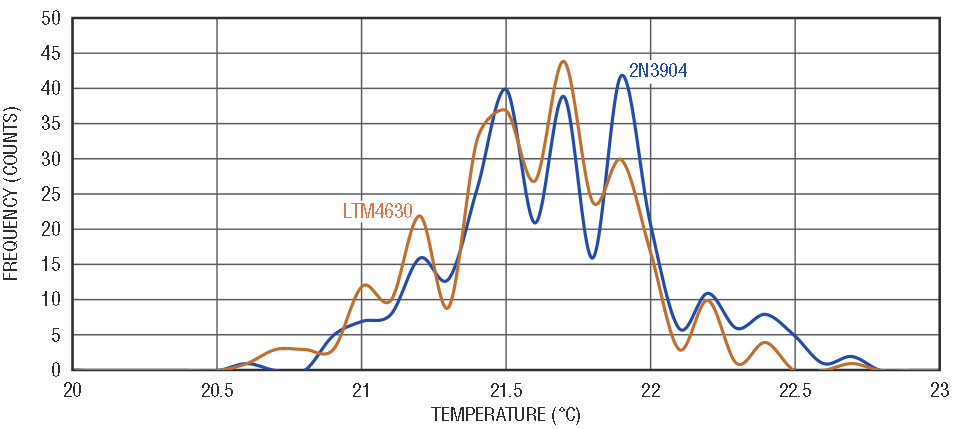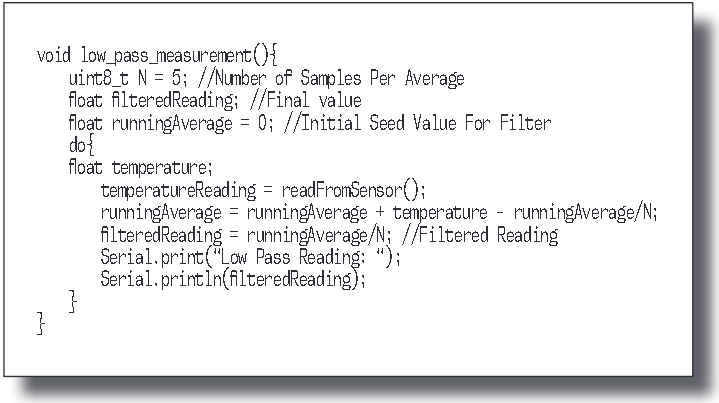# Making Accurate Temperature Measurements with Devices with Different Ideality Factors

The ability to accurately measure temperature is critical to the reliable operation of power systems. Accurate temperature data can reveal the instantaneous health of a system, and enable real-time protection functions such as thermal shutdown. For instance, significant rates of change in temperature can indicate increased power consumption. If a proper temperature feedback loop is implemented, this information can be used to reduce power consumption before temperatures escalate to dangerous levels.

Perhaps the least expensive and most ubiquitous temperature sensor is a diode. A silicon p-n junction has a forward drop of approximately 700mV and a temperature coefficient of −2.2mV/C at room temperature. This sensitivity to temperature is exploited in many systems as a means of measuring temperature by simply biasing the diode with a constant current and measuring the resulting voltage (see Figure 1).Figure 1. A resistor from 3.3V, or more, provides sufficiently constant current.

This method has achieved wide acceptance and popularity as many microprocessors, FPGAs, DC/DC converters and other high power devices include diode junctions (often in the form of bipolar transistors) for the purpose of monitoring temperature within the device itself.

Theory Of Operation

The forward voltage drop of a diode junction as a function of temperature is:(1)

where ID is the diode current, VD is the diode voltage, IS is the reverse saturation current (process dependent parameter) and η is the ideality factor (typically close to 1.0). VT is defined as:

where T is the diode junction temperature in Kelvin, k is the Boltzmann constant and q is the electron charge. VT is approximately 26mV at room temperature and scales linearly with Kelvin temperature.

IS increases with temperature, causing the ln(ID/IS) term in equation 1 to drop with temperature, yielding an approximately −2.2mV/°K composite diode voltage slope.

Unfortunately, the accuracy of these measurements depends heavily on IS, which varies from production lot to lot. Additionally, the initial offset must be calibrated out. This variability makes an accurate absolute value of temperature nearly impossible using a single point, forward voltage measurement.

As η is a constant, to obtain a linear voltage proportional to temperature the IS term is the only term that must be cancelled out. This can be achieved by measuring the change in diode voltage at two different current levels where

If

then

As

If we take a diode measurement at two different currents with a ratio of 10, the resulting voltage is 198μV per Kelvin. The absolute value of the test currents is not important, rather it is the ratio of the two currents that dictates ∆VD. This technique eliminates dependence on IS, leaving the ideality factor as the lone variable in producing a temperature from a measured voltage, where the ideality factor is essentially a constant for a given junction type, with minor variation among different junction types.

Simplify Temperature Measurements

Although the ∆VD method of measuring temperature appears simple, its use is complicated by the fact that a number of accurate data points must be collected and used in real-time calculations, namely:

• the diode is driven by two, precisely ratioed, currents
• two precise measurements of the corresponding voltages must be made
• the known current values and voltage results are recorded and the resulting temperature calculated.

Temperature monitor ICs, such as the LTC2990, LTC2991 and LTC2997 take care of these complications, while using the differential measurement technique above to eliminate errors and provide temperature measurements within 1°C of accuracy. They also compensate for series resistance errors by taking VD measurements at multiple operating currents. They measure remote and ambient temperature as well as voltage and current.

The LTC2997 provides an analog output and is ideal for overtemperature alarms and measurement. No calibration is necessary. Ratioed currents and delta voltage measurements are all made inside the part, and a voltage result is presented at the output, with a sensitivity of 4mV/°C.

Like the LTC2997, the LTC2990 and LTC2991 make all necessary measurement and temperature calculations internally. They feature four and eight measurement channels, respectively. Both devices feature an onboard ADC, which can be used to make single-ended or differential voltage measurements. They also measure temperature using an internal diode, or external, remote diodes using the measurement channels. They communicate all measurement results through an I2C interface.

Dealing with Ideality Factor

All three devices are designed to measure temperature with an accuracy of better than 1°C, using the base emitter junction of devices with an ideality factor of 1.004 such as the widely used MBBT 3904. If the ideality factor of the target sensor differs from 1.004, it can be compensated in software using the following equation(2)

It is important to consider this parameter when selecting a temperature sensor, as it could produce errors in the absolute temperature measurement. An ideality factor with a ±1% deviation from 1.004 would result in an error of 2.7°C at 0°C and an error of 4°C at 100°C. However, for most junctions, ηSENSOR error is <1% and contributes less error than device unit to unit variation.

For example, Figure 2 shows an application where the LTC2990 is interfacing with an LTM4630 μModule regulator, which has a TEMP pin with an internal PNP diode.Figure 2. Block diagram of the LTC2990 interfacing with LTM4630.

The ideality factor of this diode is 1.008, which is only a deviation of 0.4% from 1.004. As a result, absolute temperature readings from the LTM4630 while interfacing with the LTC2990 are well within 1°C of accuracy until the temperature exceeds 250°C, which is well beyond the operating range of both devices.

To demonstrate this, an experiment was conducted using the setup shown in Figure 3. A 2N3904NPN transistor was placed very close to the TEMP pin so that it would measure the same local temperature as the junction present inside the LTM4630. Both the LTC2990 and the LTM4630 unit were placed inside an oven and heated to 15°C, 25°C, 40°C, 60°C and 80°C. The LTM4630 module was powered, but no load was present so that the internal temperature of the LTM4630 does not rise above the local board temperature. Temperature measurements are made using the LTC2990’s internal sensor, the 2N3904 and the LTM4630 TEMP pin. Figure 4 shows a histogram of the measured data at 15°C.Figure 3. Test setup with a 2N3904 diode.Figure 4. Temperature measurement comparison of the LTC2990 internal sensor, a 2N3904 diode and the LTM4630 TEMP pin (connected to internal PNP diode).

The internal sensor of the LTC2990 shows the oven’s ambient temperature of approximate 15.5°C. The LTM4630 module is running a few degrees higher than the ambient temperature due to it being powered. As a result, both the 2N3904 and LTM4630 read a temperature of approximately 21.6°C. A zoomed in view of the 2N3904 and LTM4630 readings is shown in Figure 5.Figure 5. Close-up of LTC2990 temperature measurements of the 2N3904 and the LTM4630.

The correlation between the two sensors is easily seen as the distribution centers around 21.8°C for both sensors and is within 1°C of each other, which validates our previous claim of the error being within 1°C of each other due to the ideality factor deviation of only 0.4%. No correction for η is necessary.

Oversampling for Better Resolution

The widths of the curves represent the ADC quantization noise, which can easily be filtered out through software. While filtering can be done using a running average, it requires the processor to store data over the number of samples being averaged. For processors with memory constraints this might not be desirable. Figure 6 shows the pseudocode used to implement a lowpass filter.Figure 6. C psuedocode implementation of lowpass filtering. Sample code available here.

Filtering is done by adding new temperature data and then subtracting the mean each time. The advantage of this technique is that the history of data need not be stored. If N is a power of 2, the division can be accomplished by a simple shift. Readings were taken again, with Figure 7 showing the filtered data distribution. A code example using the DC2026 Linduino® along with the LTC2990 is available here.Figure 7. Filtered LTC2990 temperature measurement comparison between 2N3904 And LT4630.

Notice how the temperature peaks are offset by 0.1°C. This error is due to the ideality factor error of 0.4%. If we compensate for the ideality factor using Equation 2, the two peaks line up nicely, as shown by Figure 8. Compensation ensures that the sensors with different η values can produce the same absolute temperature under the same conditions.Figure 8. LTC2990 temperature measurement comparison between a 2N3904 and a software-calibrated LTM4630.

Summary

Our temperature monitors make temperature measurements simple and convenient. Measurements are independent of reverse saturation current and series resistance. Errors introduced by interfacing with devices of different ideality factors, while often negligible, can be precisely calibrated, allowing use of temperature monitors with a wide variety of diode temperature sensors.